Mobile QR Code1. (Dept. of Electrical and Computer Eng., Inha University, 100, Inha-ro, Michuhol-gu, Incheon, Incheon 22212, Korea)

Spread spectrum clock generator (SSCG), dual-tone modulation profile, Hershey-Kiss modulation profile

## I. INTRODUCTION

As the data rate increases in serial interfaces, a higher electromagnetic interference (EMI) level is becoming an issue for system design. There are various approaches to reduce EMI levels such as shielding, slew-rate control, low voltage differential clocking, pulse shaping, and spread spectrum clocking (SSC) [1-4]. Among them, the spread spectrum clocking method is a simple and effective way to reduce the EMI level . The spread spectrum clock generator (SSCG) is a phase-locked loop (PLL)-based clock generator that spreads the output clock signal energy concentrated in a narrow band into a wider band spectrum. The modulation profile of an SSCG has a direct effect on the spectrum spreading of the output frequency. Thus, an optimal modulation profile is needed to reduce EMI reduction as much as possible . In SSCG circuits, a triangle modulation profile is mostly adopted for its ease of implementation. However, because most harmonic energies in the triangular modulation profile are present on the edges of the spectral distribution, EMI reduction is not optimal. Until now, a variety of design approaches for SSCG circuits with different types of modulation profiles have been reported [3-15]. They mostly utilize a triangular modulation technique. It is known that a non-linear Hershey-Kiss modulation profile gives more uniform spectrum distribution, and that more EMI reduction can be achieved than with a triangular modulation profile. Implementation of the nonlinear Hershey-Kiss modulation profile SSCG usually requires a somewhat complicated design approach such as a look-up table approach  or a frequency-locked loop (FLL)-based SSCG with digital-to-analog converters (DAC) and a delta-sigma modulator (DSM) . Another SSCG with a nonlinear Hershey-Kiss profile was realized utilizing dual DSMs . In , the Hersey-Kiss modulation profile was obtained by connecting two up/down counters and dual DSMs in series. The low EMI reduction of 15dB was measured in . This is due to a large clock jitter induced by quantization noise from two DSMs in series and an increased spectrum density near the center frequency during modulation from the first order DSM.

In this paper, a revised approach is proposed to generate a Hershey-Kiss modulation profile using two up/down counters and a single delta-sigma modulator. The first order DSM for the slope control in  was removed, by which evenly distributed the spectral power density can be achieved. This leads to a better EMI reduction. Adjustments of all design variables associated with the proposed SSCG can be done digitally, thus the proposed SSCG has enhanced portability for various applications and specifications. Additionally, a dual-tone modulation profile was adopted to reduce further the EMI .

This paper is composed of five sections. In Section II, the design principle of the proposed Hershey-Kiss modulation profile is explained. The setting of the design variables and circuit design are described in detail in Section III. The measurement results are given in Section IV and the final conclusions are presented in Section V.

## II. HERSHEY-KISS PROFILE GENERATION METHODOLOGY

Fig. 1 shows a frequency output with a typical Hershey-Kiss modulation profile based a center spreading case. The output frequency variation vs. time with the Hersey-Kiss modulation profile is shown in Fig. 1(a). The frequency slope over time ($df/dt$, ts time derivative) in Hershey-Kiss modulation profile can be approximated as a linear curve as shown in Fig. 1(b). The frequency slope can be linearly modeled, and it has the lowest value at the center frequency and the highest value at the maximally spread frequencies. Since the frequency slope curve vs. time is represented as a linearized continuous curve (shown in Fig. 1(b)), it can be discretized over multiple time steps with a fixed time interval as shown in Fig. 1(c) for processing digitally. If the slope variation is represented with more numbers of discrete timing interval steps, the better Hershey-Kiss profile approximation can be represented. Each time interval is illustrated as a dotted line in the curves. Therefore, a Hershey-Kiss modulation profile can be generated by a linear discrete frequency slope change over time with alternative toggling of positive and negative slope and periodic repeating.

In summary, if the discrete frequency slope is properly controlled over time intervals, the Hershey-Kiss modulation profile can be generated. This is the design principle of the proposed SSCG. In addition, the proposed SSCG is built with a digital-based modulation controller so that various design variables can be set digitally as described in section III. The digital controllability of the design parameters enhances the design portability and flexibility.

##### Fig. 1. Frequency output with a typical Hershey-Kiss modulation profile: (a) Frequency output variation vs. time; (b) Linearly approximated continuous frequency slope vs. time; (c) Discrete frequency slope approximation over discrete time intervals (dotted lines) vs. time.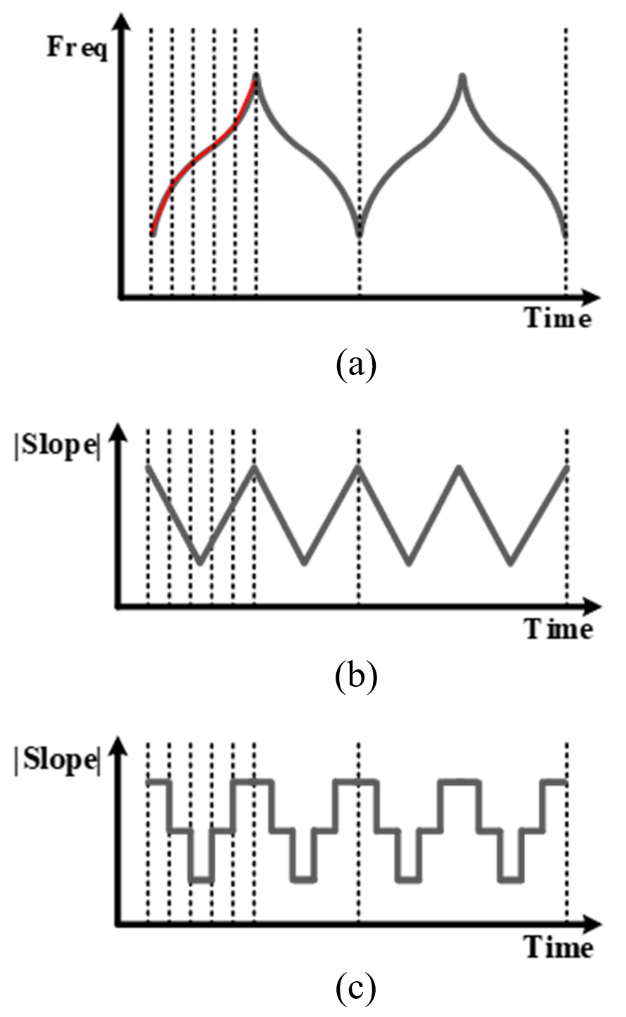## III. THE PROPOSED SSCG CIRCUIT DESIGN

Fig. 2 shows a block diagram of the proposed SSCG, which is composed of a phase-locked loop (PLL) and a digital modulation controller.

The PLL is a fractional-N PLL consisting of a phase-frequency detector (PFD), a charge-pump (CP), a loop filter (LF), a voltage-controlled oscillator (VCO), and a multi-modulus divider (MMD). The modulation controller consists of two Hershey-Kiss profile generators (1 and 2) for dual-tone operation and a single delta-sigma modulator (DSM). The DSM output is provided to the MMD to change the division ratio of the PLL periodically. By varying the division ratio of the MMD, the output frequency is modulated and spread. The dual-tone generator mixes outputs from two different profile generators. The main difference from previous SSCG architectures is that the proposed SSCG is modulated with Hershey-Kiss profile for achieving a better EMI reduction.

##### Fig. 2. Block diagram of the proposed SSCG.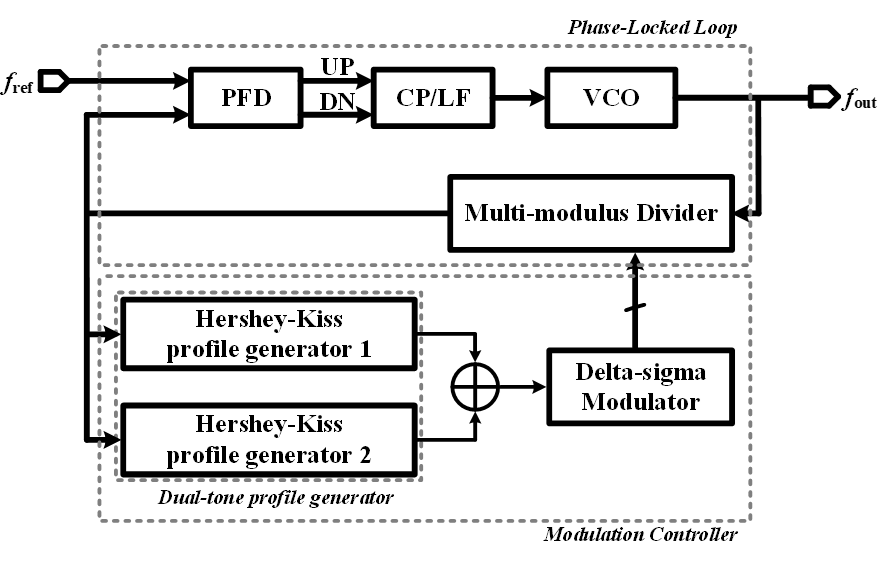### 1. Proposed Hershey-Kiss Profile Generator

Fig. 3 shows a block diagram of the proposed Hershey-Kiss profile generator. The slope profile shown in Fig. 1(c) is to be generated. The profile generator has two UP/DN counters and accepts five input signals to control the modulation parameters. The input signals include the initial value of the slope (IS), number of discrete steps for slope control (M), change of the slope per counting step (DS), peak counting value (K), and the spreading type select (STS: down (‘01’), center (‘10’), up spreading (‘11’)). The outputs are a sign (either positive or negative) and a slope value ($Out\_ slope$), which are provided to the DSM. After initializing the output slope of the profile generator with IS, UP/DN_counter_1 and UP/DN_counter_2 begin to operate. The peak counting value of K sets the output range of the UP/DN_counter_1 and the counter output counts up and down from ‘0’ to K and K to ‘0’, repetitively. The period of the counting value profile relates to the targeted modulation frequency.

Fig. 4(a) shows the generation process of the proposed Hershey-Kiss frequency profile. The profile generator produces a slope (Out_slope) having stairs-like discrete step patterns (moving up or down) through UP/DN_counter_2 as the output of UP/DN_counter_1 changes from ‘0’ to K, and vice versa, repetitively. UP/DN_counter_2 is for generating the number of discrete steps of slope change over a quarter period of the modulation frequency. If we use the more discrete steps for a linear frequency slope change over a given period, the slope change on each discrete step becomes smaller. The number of discrete steps of the slope can be adjusted by the external input M. UP/DN_counter_2 produces a new pulse for every K/M pulse of the UP/DN_counter_1. By these newly produced pulses, M different output slope values with step values (DS) are generated during a quarter period of the modulation frequency. Fig. 4 shows the example of Out_slope when M is set as 5. The profile generator is not to make more than M slope values when the counting value of UP/DN_counter_1 reaches K or ‘0’. The sign value gives a positive or negative slope and is toggled whenever the counter output value reaches K. When the value of the sign output is ‘0’, the slope of the modulation frequency is positive, and the output frequency increases. When the value of the sign output is ‘1’, the slope is negative, and the output frequency decreases. If the sign output is ‘0’, the output frequency increases, and its absolute slope is gradually decreased from the maximum to the minimum. Once the slope reaches the minimum, then it is gradually increased from the minimum to the maximum. When the counter output reaches K, sign switches to ‘1’ and the output frequency decreases. Its absolute frequency slope is gradually decreased from the maximum to the minimum. Once the slope reaches the minimum, then the slope increases from the minimum to the maximum. The generated slope output makes the frequency variation smaller at the center frequency and larger at the minimum and maximum frequencies over time. As a result, the output frequency created is a Hershey-Kiss profile, as shown at the bottom of Fig. 4(a). The continuous profile of the output frequency is shown with a black line. Based on the discrete step value of Out_slope, the final profile is approximated as a piecewise linear curve with a red line at the bottom (see Fig. 4(a)).

One period of the modulation profile consists of a positive slope swing and negative slope swing. The shape of generated VCO control voltage is the same as the modulation profile. Consequently, the period of UP/DN_counter_1 is the same as the half-period of the sign output of the profile generator and the modulation frequency ($f_{m}$: modulation frequency) is given by Eq. (1),

##### (1)
$f_{m}=\frac{f_{ref}}{4K}$

, where K is a peak counting value. If K is represented by a 10-bit counter and the targeted modulation frequency is 33 kHz with a reference clock of 90 MHz, then K can be set as 682. If the target modulation frequency is 30 kHz, K is set as 750.

As shown in Fig. 4(b), if the slope output (Out_slope) does not change (DS = 0), a typical triangular modulation profile is generated because the frequency slope is constant. Proper IS and DS values can be set on targeted spreading parameters of the SSCG. The maximum variation range of the slope output value (D$_{M}$: | max. value – min. value | of Out_slope) during a half-period of the modulation profile is given by Eq. (2).

##### (2)
$D_{M}=2\times \frac{K}{M}\times \sum _{j=0}^{M-1}\left(IS-j\times DS\right)$

The value (D$_{M}$) is provided to the input of the DSM. Because two Hershey-Kiss profile generators were implemented, both D$_{M1}$ from the profile generator 1 and D$_{M2}$ from the profile generator 2 are utilized for dual-tone mode operation. The 2-bit-size input signal (spread type select: STS) decides one of three spreading types, which are down, center, or up spreading. When the external signal is ‘00’, ‘01’, ‘10’, or ‘11’, the circuit operates in non-SSC, down-spread, center-spread, or up-spread modes, respectively, as shown in Fig. 5.

##### Fig. 3. Proposed Hershey-Kiss profile generator.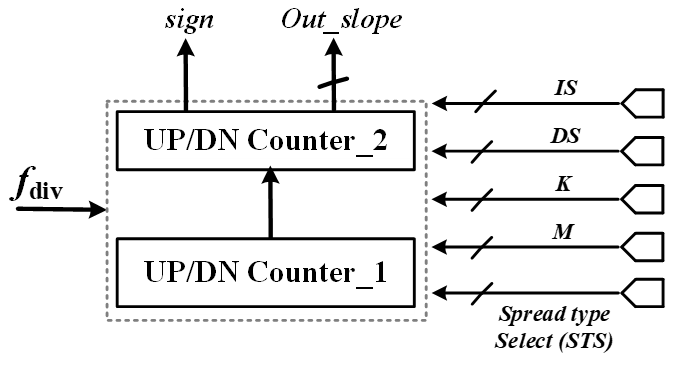##### Fig. 4. (a) Generation of the proposed Hershey-Kiss modulation profile (black line in Output frequency: continuous $Out\_ slope$, red line in Output frequency: discrete $Out\_ slope$); (b) Typical triangular modulation profile generation.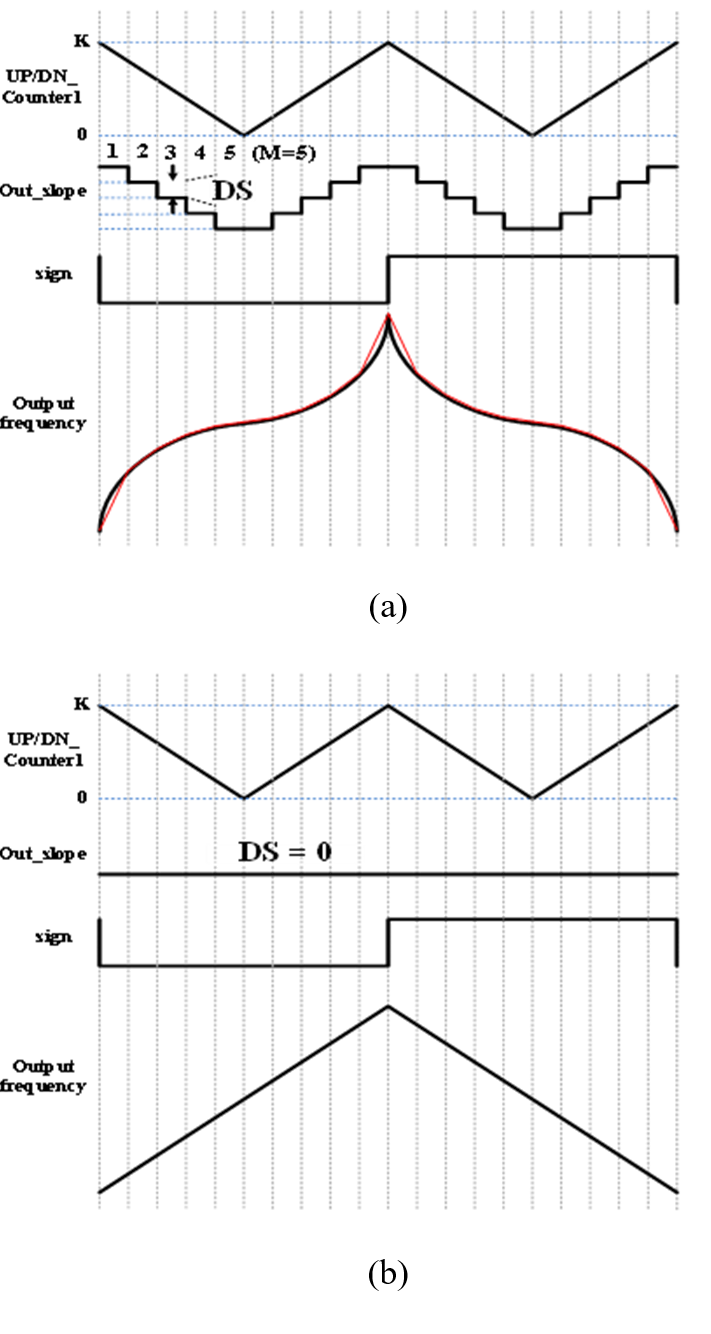##### Fig. 5. (a) Non-SSC (STS = 00); (b) Down spreading (STS = 01); (c) Center spreading (STS = 10); (d) Up spreading (STS = 11).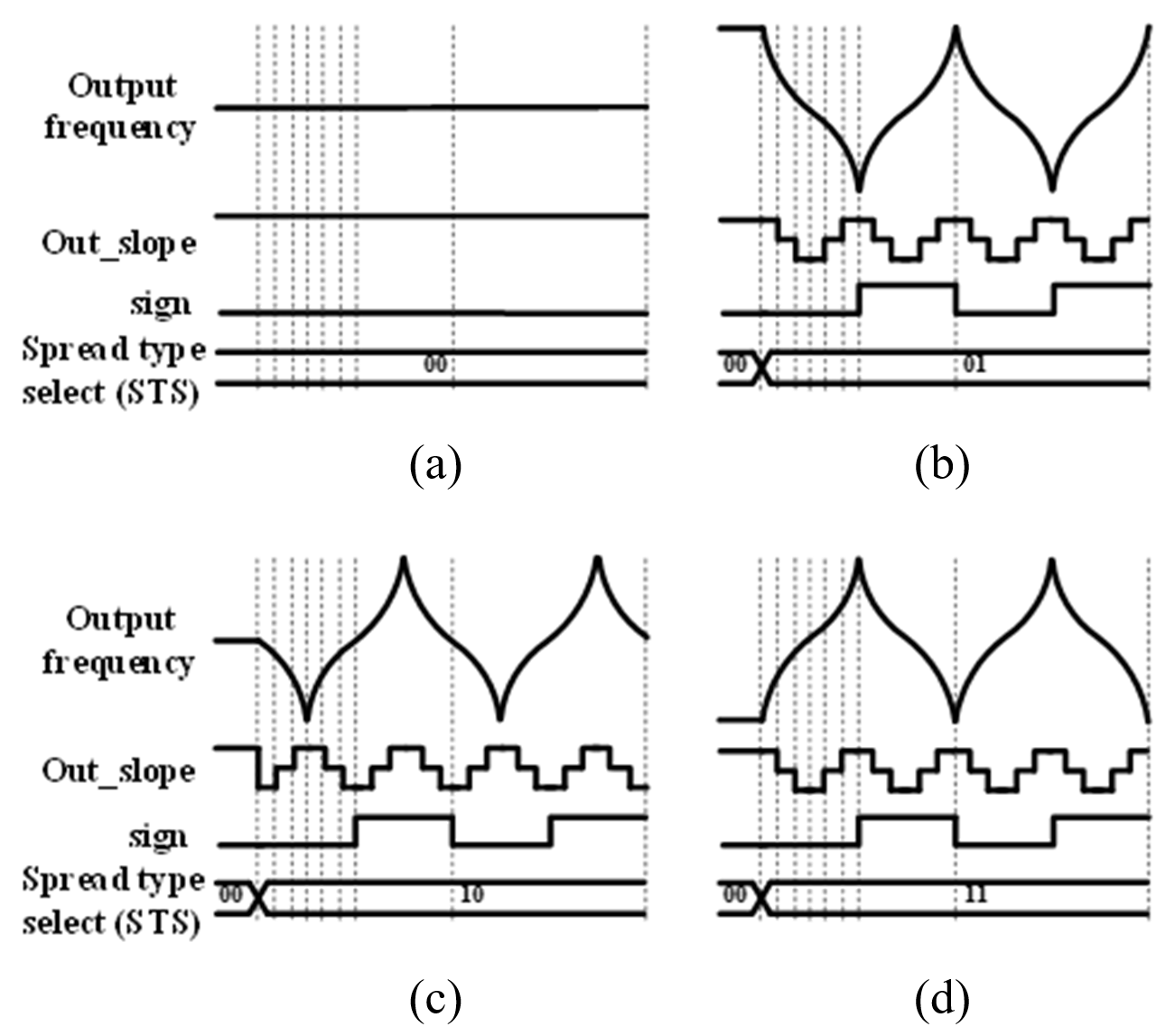### 2. Delta-sigma Modulator (DSM)

The delta-sigma modulator used in the proposed structure is the 1-1 structure 2$^{\mathrm{nd}}$ order multi-stage noise shaping (MASH) delta-sigma modulator. To optimize between randomization and quantization noise effect of DSM, the second order DSM was adopted. The DSM of 2$^{\mathrm{nd}}$ order MASH structure is stable. If a higher order DSM were adopted, the phase jitter of the output clock could be increased.

Fig. 6 shows the MASH 1-1 DSM structure that takes outputs from the 15-bit Hershey-Kiss profile generator output and produces 4-bit output. The 4-bit output of the DSM is provided to the multi-modulus divider (MMD) in the PLL. The DSM generates three overflow bits (C0, C1, and C2), which are mapped onto the 4-bit signal for MMD. As the overflow bits in the modulator change, the average output of the DSM changes. Through the modulus mapping circuit shown in Fig. 6, the values of C0, C1, and C2 are mapped onto 4 bits (R0, R1, R2, and R3) that are provided to the multi-modulus divider. Transfer function of the DSM can be derived as follows . The overflow bit from a single accumulator with a feedback delay ($z^{-1})$ can be expressed

##### (3)
$C_{0}\left[z\right]=\frac{\frac{1}{\left(1-z^{-1}\right)}}{1+\frac{z^{-1}}{\left(1-z^{-1}\right)}}X\left[z\right]+\frac{1}{1+\frac{z^{-1}}{\left(1-z^{-1}\right)}}e_{1}\left[z\right]\\ =X\left[z\right]+\left(1-z^{-1}\right)e_{1}\left[z\right]$

Similarly, $C_{1}\left[z\right]=-e_{1}\left[z\right]+\left(1-z^{-1}\right)e_{2}\left[z\right]$ and $C_{2}\left[z\right]=-z^{-1}C_{1}\left[z\right]$. Therefore, $Y\left[z\right]$ can be derived as

##### (4)
$Y\left[z\right]=C_{0}\left[z\right]+C_{1}\left[z\right]+C_{2}\left[z\right]\\ =e_{2}\left[z\right]\left(1-z^{-1}\right)^{2}+X\left[z\right]$

Therefore, the signal transfer function (STF) and the noise transfer function (NTF) given as Eqs. (5) and (6), respectively.

##### (5)
$STF\left(z\right)=1$
##### (6)
$NTF\left(z\right)=\left(1-z^{-1}\right)^{2}$

Table 1 shows an example of generation of the division ratio $N~$between 54 and 57 by the 4-bit mapped outputs (R1, R2, R3, and R4) of DSM. Fig. 7 shows the MMD block diagram.

When the targeted spreading ratio (${\delta}$) is given, the value of ${\Delta}$N can be derived. Once ${\Delta}$N is determined, the value of $D_{in}$ can be derived with a given DSM bit-size. The relationship between the VCO output frequency and the reference input frequency can be expressed as Eq. (7).

##### (7)
$F_{VCO}=F_{REF}\times \left(N+\Delta N\right)$

, where N is the targeted integer division ratio and ${\Delta}$N is the fractional division ratio of the PLL. These are related to the targeted spread ratio of the SSCG. The fractional division ratio ($\Delta N$) is the average value of the DSM output and can be expressed by Eq. (8).

##### (8)
$\Delta N=average\,\,output\,\,from\,\,DSM \\ =\frac{D_{in}}{2^{n}}=\frac{D_{in}}{2^{DSM\_ bit\_ size}}$

, where $D_{in}~$is a DSM input. As shown in Eq. (6), if ${\Delta}$N is determined from a given spreading ratio (${\delta}$), the value of $D_{in}$ can be obtained from Eq. (8). The procedure of setting design variables is explained in detail with an example in section III-5.

##### Fig. 6. Second order MASH 1-1 delta-sigma modulator.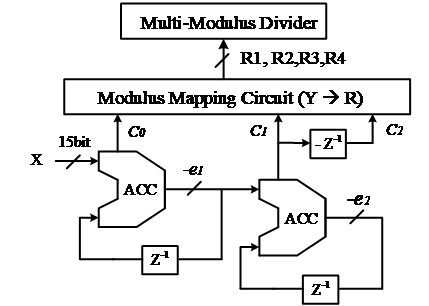##### Fig. 7. Block diagram of the multi-modulus divider (MMD).}##### Table 1. Modulus mapping circuit for N (55~57)
 C0 C1 C2 R0 R1 R2 R3 N 0 0 0 1 1 1 0 55 0 0 1 0 1 1 0 54 0 1 0 0 0 0 1 56 0 1 1 1 1 1 0 55 1 0 0 0 0 0 1 56 1 0 1 1 1 1 0 55 1 1 0 1 0 0 1 57 1 1 1 0 0 0 1 56

### 3. Spread Ratio and Design Parameters

As shown in the previous section, the maximum variation of the output value ($D_{M}$) during the half cycle of the modulation profile is given as Eq. (2). Now, the relationship between design parameters and the targeted spreading ratio is described. The spreading ratio (${\delta}$) can be expressed as in Eq. (9), where N is the targeted integer division ratio and ${\Delta}$N is the fractional division ratio of PLL, which is related to the spread ratio.

##### (9)
$\delta =\frac{\Delta f}{f_{1}}=\frac{f_{1}-f_{2}}{f_{1}}=\frac{f_{REF}\times \left(N+\Delta N_{1}-N-\Delta N_{2}\right)}{f_{REF}\times \left(N+\Delta N_{1}\right)}=\frac{\Delta N_{1}-\Delta N_{2}}{N+\Delta N_{1}}$

Therefore, substituting Eq. (8) into Eq. (9), the spreading ratio (${\delta}$) can be rewritten as Eq. (10).

##### (10)
$\delta =\frac{\frac{\left| D_{1}-D_{2}\right| }{2^{DSM\_ bit\_ size}}}{N+\frac{D_{1}}{2^{DSM\_ bit\_ size}}}=\frac{\left| D_{1}-D_{2}\right| }{N\times 2^{DSM\_ bit\_ size}+D_{1}},$

, where $\left| D_{1}-D_{2}\right|$ is equal to the maximum variation of the slope output value of the profile generator. Thus, $\left| D_{1}-D_{2}\right|$=$D_{\mathrm{M}}$. For the targeted spread ratio, $D_{1}$ is set to an appropriate value. Therefore, in the case of a single-tone modulator, the spread ratio can be written as in Eq. (11).

##### (11)
$\delta _{signle-tone}=\frac{\left| D_{M}\right| }{N\times 2^{DSM\_ bit\_ size}+D_{1}}$

In case a dual-tone profile generator is used, the spread ratio is expressed as Eq. (12).

##### (12)
$\delta _{dual-tone}=\frac{\left| D_{M1}\right| +|D_{M2}|}{N\times 2^{DSM\_ bit\_ size}+D_{1}}$

Because the slope output variation $\left(D_{M}\right)$ is determined by the initial slope (IS), delta slope (DS), M value, and bit-size of DSM (as given in Eq. (2)), the spread ratio (${\delta}$) can also be adjusted with values of IS, DS, M, and the DSM bit-size.

### 4. Dual-tone Modulation

As the spreading ratio increases, the frequency variation increases, and the EMI reduction effect is increased. However, as the spread ratio increases, output clock jitter also increases. As a result, this gives a design the complexity of having a clock and data recovery circuit (CDR) in the receiver. If a single-tone modulation profile is used with a fixed spreading ratio, the spectrum appears at the same interval as an integer multiple of the modulation frequency. To reduce EMI, the frequency spacing at which the spectrum appears needs to be reduced. A dual-tone modulation profile is a way to reduce EMI further . The dual-tone modulator is implemented by adding outputs from two profile generators as shown in Fig. 2. The dual-tone modulators produce two different profiles of frequencies as shown in Fig. 8. Due to the mixing of a low frequency component that changes the amplitude of the output signal, the final spectral interval is not uniform. Thus, the spectral energy is dispersed in a narrower frequency interval. As a result, a further EMI reduction can be achieved. Ref.  produced a dual-tone profile with triangular shapes and achieves 18.8 dB EMI reduction. The proposed SSCG approach adopted the dual-tone modulation approach from Ref. . However, dual-tone Hershey-Kiss modulation technique instead of dual-tone triangular modulation was applied and enables to achieve 28.7 dB EMI reduction. The combination ratio (1-${\alpha}$, ${\alpha}$) of the two tones is made up by an appropriate combination of peak values as shown in Fig. 8. The optimal ${\alpha}$ value was calculated to be about 0.86 . Thus, when in dual-mode profile operation, the mixing ratio of 0.86 is used in the proposed SSCG.

##### Fig. 8. Dual-tone modulation profiles.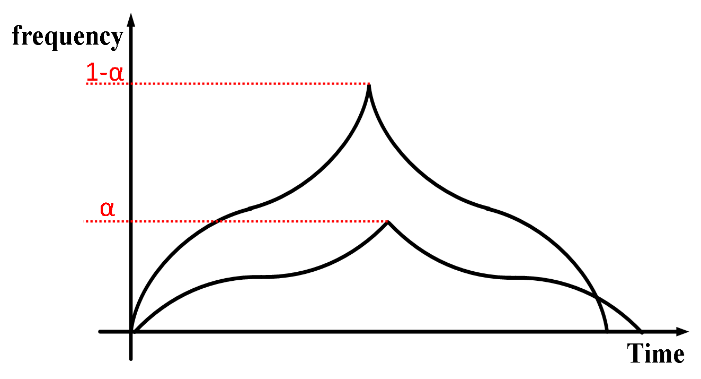### 5. Procedure for Setting the Design Parameters

In this section, the procedure for setting the SSCG design parameters is described. First, assume that a 5~GHz output clock frequency is required with a 90 MHz input reference clock. Then from Eq. (7), we can derive N = 55 and ${\Delta}$N = 0.5556, if an SSCG having a modulation frequency of 33 kHz and a spread ratio of 0.5% is to be designed with a single-tone profile generator. With a 15-bit DSM, $2^{SDM\_ bit\_ size}=2^{15}=32768$. Using Eqs. (7) and (8), the design variable of $D_{1}$, which is the maximum value of DSM input can be derived as 18,205 with ${\Delta}$N = 0.5556. By substituting the modulation frequency of 33 kHz in Eq. (1), the peak counting value of UP/DN_counter1, $K_{1}$= 682. As M value is increased, smoother modulation profile and a better EMI reduction is expected. Through simulations with various M values, EMI reduction on different M values has been observed. The simulations showed that little EMI reduction improvement has been achieved when M is more than 5. Thus, M is set as 5 in this design. If we increase DS as 2, then IS value is determined as 11. However, as the DS value goes higher, frequency variation becomes smaller near the center frequency, so EMI reduction becomes smaller. Therefore, for more even distribution of frequency variation, we chose DS = 1 in the proposed SSCG design. Under various design constraints, IS and DS values can be optimized for best EMI reduction through simulations. Setting $M_{1}$= 5, $DS_{1}$= 1, and $IS_{1}$= 9, the maximum variation of the profile generator, $D_{M1}$, is calculated as 9520. From this, by plugging those values into Eq. (11), the targeted spread ratio of 0.5% is obtained as shown Eq. (13).

##### (13)
$\delta _{single-tone}=\frac{9520}{55\times 2^{15}+18205}\approx 0.5\mathrm{\% }$

In case the dual-tone profile generator is applied, the second modulation frequency of 30 kHz is added. The values of $K_{1}$ and $K_{2}$ are obtained using Eq. (1) at the given modulation frequency. From $f_{m2}=\frac{fref}{4\times K_{2}}=$ $30kHz$, $K_{2}$ becomes 750 and $K_{1}$ is 682 at a modulation frequency of 33 kHz as before. The other design variables are set as $IS_{1}$= 8, $DS_{1}$= 1, $M_{2}$= 3, $IS_{2}$= 2, and $DS_{2}$= 1 with the 15-bit DSM. The $IS_{1}$value fell from 9 (in a single-tone profile generator case) to 8, so that the $\left| D_{M1}\right| +|D_{M2}|$ value was adjusted at near 9600 for a 0.5% spread ratio. From the predetermined design variable values, $D_{M1}$= 8160 and $D_{M2}$= 1500 can be obtained. Therefore, in the case of the dual-tone profile generator, the final spread ratio $\delta _{dual-tone}=\frac{9660}{55\times 2^{15}+18205}\approx 0.5\mathrm{\% }\,,$which meets the targeted spread value.

## IV. EXPERIMENTAL RESULTS

The simulation has been done with a 65 nm CMOS process on Hershey-Kiss modulation profile generation. The design target is for generating the output clock with a center frequency of 5 GHz using a 90 MHz input reference clock. The SSCG with modulation frequencies of 33 kHz and 30 kHz and a target spread ratio of 0.5% was designed and simulated. The design parameter settings applied to the SSCG circuit design are described in section III-5. Fig. 9 presents a simulation result showing the single-tone Hershey-Kiss modulation profiles of three spreading types. When the input STS values are ‘01’, ‘10’, and ‘11’, the down-spread (Fig. 9(a)), center-spread (Fig. 9(b)), and up-spread (Fig. 9(c)) modes are shown, respectively. The simulation results of a single-tone modulation profile (Fig. 10(a)) and dual-tone modulation profile (Fig. 10(b)) with a 0.5% spread ratio are also shown, respectively. In a single-tone modulation case, the positive or negative peak value is constant over time. However, in dual-tone modulation mode, the peak values are varied due to mixing two different modulation frequencies. To show the difference between a single-tone modulation profile and a dual-tone modulation profile, the x-axis scales are different in Fig. 10(a) and (b). The simulated phase noise is shown in Fig. 11. Our SSCG has a -92 dBc/Hz phase noise at 5 GHz with a 1 MHz offset.

To verify the proposed SSCG circuit, it has been fabricated using a 65 nm CMOS process. Fig. 12 shows the layout of the proposed SSCG circuit and the chip image. The size of the core block occupies an area of 231 ${\times}$ 191 ${\mu}$m, and the entire circuit area including the loop filter is 735 ${\times}$ 530 ${\mu}$m. The following presents measurement results of the proposed SSCG circuit with the design parameters in Section III-5. Fig. 13 shows the measured frequency spectra at the output frequency of 5 GHz when operating in single-tone profile mode. The resolution bandwidth (RBW) of the frequency analyzer was set to 10 kHz.

The measurement results show that the peak power level of the SSC is reduced by 24.6 dB in down-spread mode, 24.4 dB in center-spread mode, and 23.9 dB in up-spread mode, with a 0.5% spreading ratio. Fig. 14 shows the measured EMI reduction of the proposed Hershey-Kiss single-tone profile and the dual-tone profile case. When operating in dual-tone mode, peak power reduction of 28.7 dB is achieved. An additional EMI reduction of 4.1~dB was achieved in dual-tone mode case compared to single-tone mode. Fig. 15 shows the measured output clock signal modulated with the dual-tone Hershey-Kiss modulation. The rms jitter and peak-to-peak jitter of the output clock are 2.39 ps and 16.4 ps, respectively.

Table 2 shows the circuit performance summary of the proposed SSCG design and comparison with other recently published SSCG designs. The key performance is EMI reduction. The performance comparison shows that the proposed SSCG circuit with dual-tone Hershey-Kiss modulation has a better EMI reduction (28.7 dB) than in other SSCG work published recently. In most of the SSCG works, EMI reduction measurements were done with a 10 kHz RBW. In terms of chip area, compared to Ref.  and Ref.  fabricated with the same 65 nm CMOS process, digital blocks for the dual modulation profile generators. If loop filter of the proposed SSCG occupying about 85% of the chip area and the placement of the other blocks were optimized, the chip area could be reduced. The proposed SSCG chip consumes 8.5 mW for 5GHz clock generation with dual-tone modulation operation and achieves 28.7 dB EMI reduction. Ref.  using the same process shows a 6.34 mW power consumption for 3.2 GHz clock and achieves only 18.5 dB EMI reduction. And Ref.  generating the same 5GHz clock consumes 9 mW with 26~dB EMI reduction.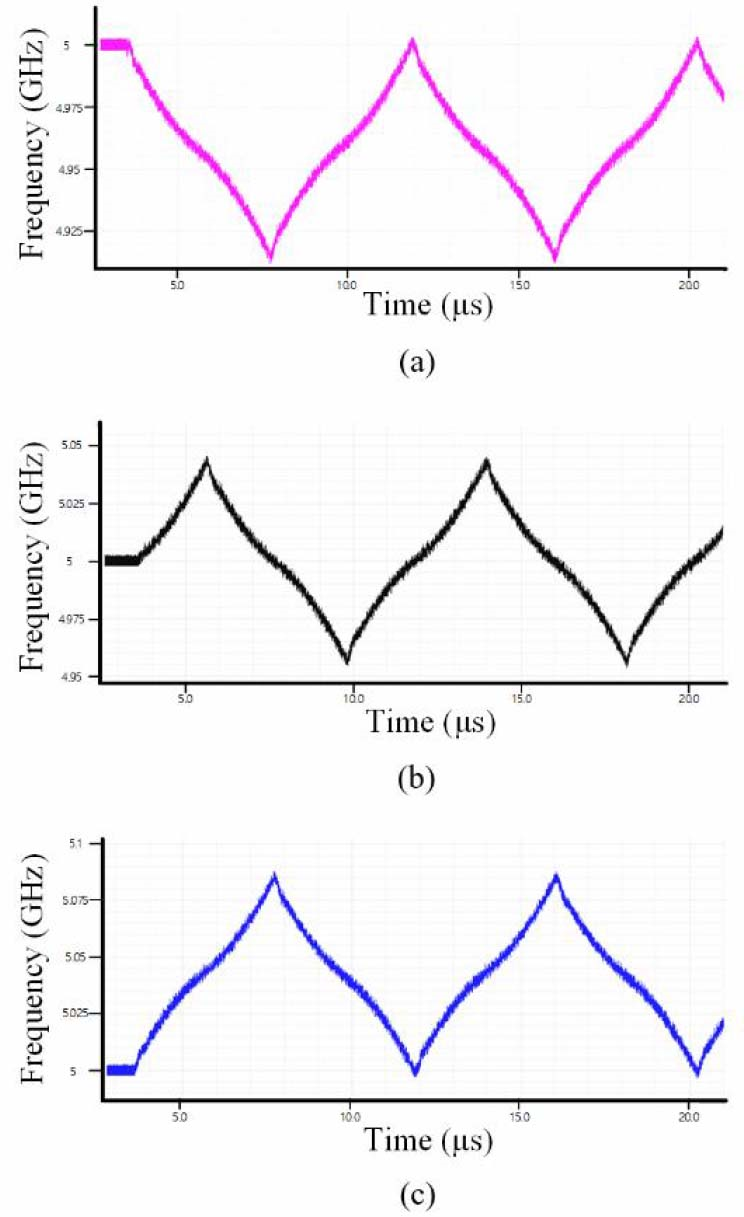##### Fig. 10. Simulated modulation profile: (a) Single-tone modulation case; (b) Dual-tone modulation case (0.5% spreading ratio).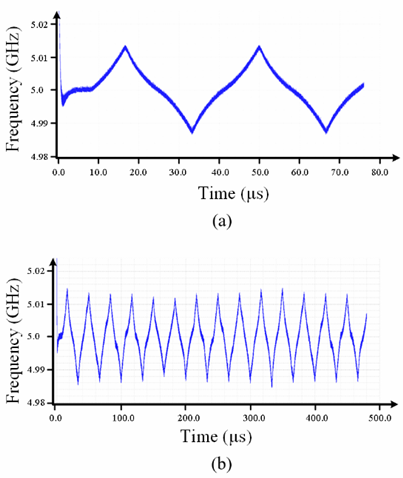##### Fig. 11. The simulated phase noise at 5 GHz with frequency offsets of 10 kHz, 100 kHz, 1 MHz and 10 MHz.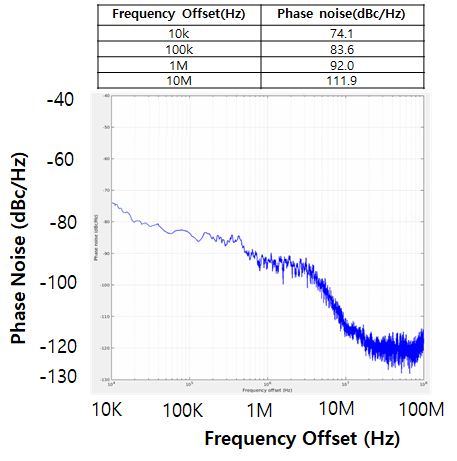##### Fig. 12. (a) Chip photograph; (b) Layout.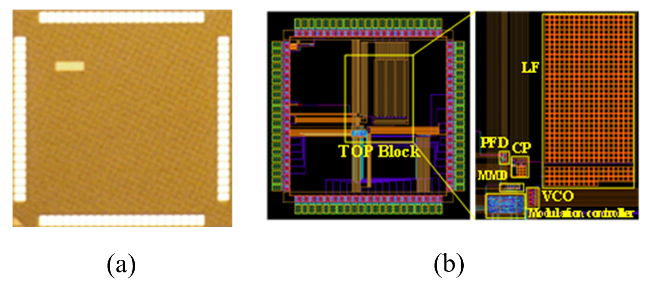##### Fig. 13. Measured output spectra in three different spread types with single-tone modulation: (a) Non-SSC; (b) Down spreading; (c) Center spreading; (d) Up spreading.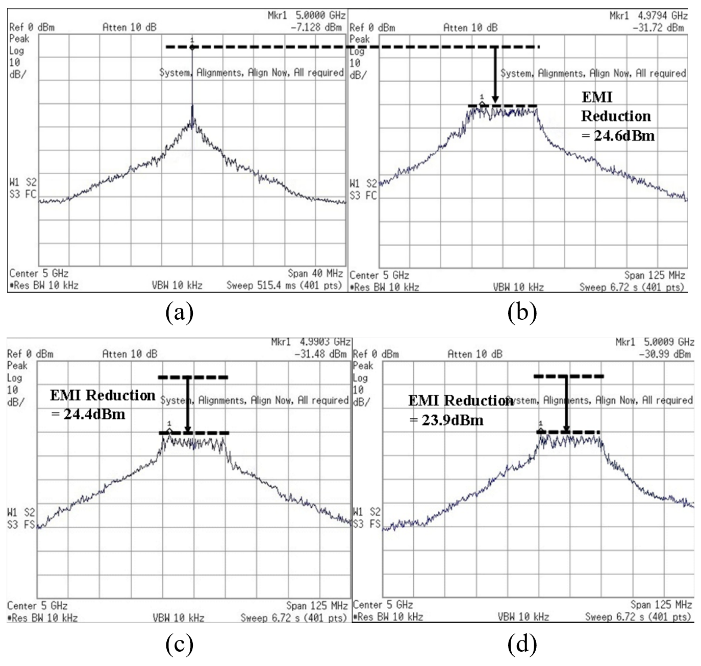##### Fig. 14. Measured output spectra: (a) single-tone case; (b) dual-tone case.}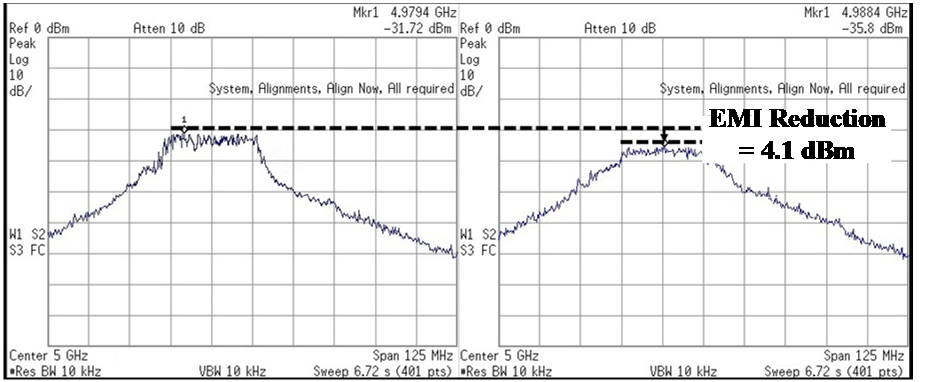##### Fig. 15. Measured clock jitter with dual-tone modulation.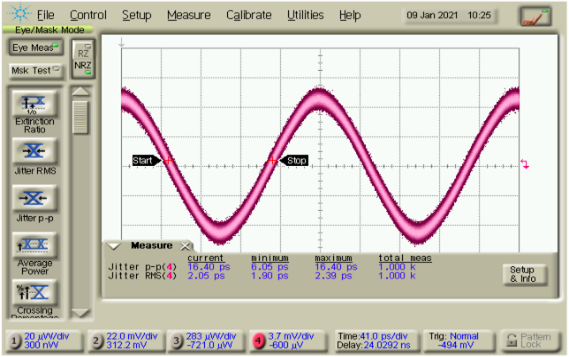##### Table 2. Performance summary and comparison with previous work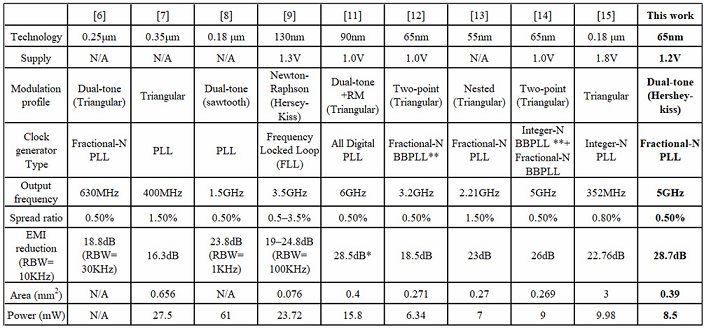## V. CONCLUSIONS

In this paper, a SSCG circuit using a dual-tone modulation Hershey-Kiss profile is presented. The targeted spread ratio was 0.5% with dual modulation frequencies of 33 and 30 kHz. The design parameters can be adjusted digitally as the design specifications change. The proposed SSCG showed 28.7 dB of EMI attenuation as measured with an RBW of 10 kHz. Compared to the single-tone modulation case, 4.1 dB of additional EMI reduction was achieved.

## ACKNOWLEDGMENTS

This work was supported by Inha University. CAD tools and chip fabrication were provided by IDEC.

## References

1
J. H. Kim, et al., “Dithered timing spread spectrum clock generation for reduction of electromagnetic radiated emission from high-speed digital system,” Electromagnetic Compatibility, IEEE International Symposium on, Vol. 1, pp. 413-418, Aug., 2002.2
K. B. Hardin, et. al., “Spread spectrum clock generation for the reduction of radiated emissions,” Electromagnetic Compatibility, Proceedings of IEEE Symposium on, pp. 227-231, Aug., 1994.3
K. Hardin, et al., “Investigation into the Interference Potential of Spread-Spectrum Clock Generation to Broadband Digital Communica-tions,” Electro-magnetic Compatibility, IEEE Transactions on, Vol. 45, No. 1, pp. 10-21, Feb., 2003.4
Nurcan Keskin, Huaping Liu, “Practical Considerations for Electromagnetic Interference Suppression Rate with Spread Spectrum Clocking,” Compatibility Magazine, IEEE Electromagnetic, Vol. 5, No. 2, pp. 57-60, Aug., 2016.5
S. W. Oh, et al., “A Spread Spectrum Clock Generator for DisplayPort 1.2 with a Hershey-kiss Modulation profile,” semiconductor technology and science, Journal of, Vol. 13, No. 4, pp. 282-290, Aug., 2013.6
Deok-Soo Kim and Deog-Kyoon Jeong, "A spread spectrum clock generation PLL with dual-tone modulation profile," VLSI Circuits Digest of Technical Papers., 2005 Symposium on, pp. 96-99, Jun., 2005.7
Y. Hsieh and Y. Kao, “A Fully Integrated Spread-Spectrum Clock Generator by Using Direct VCO Modulation,” Circuits and Systems I: Regular Papers, IEEE Transactions on, Vol. 55, No. 7, pp. 1845-1853, Aug., 2008.8
J. Lee, et al., “Spread spectrum clock generation for reduced electro-magnetic interference in consumer electronics devices,” Consumer Electronics, IEEE Transactions on, Vol. 56, No. 2, pp. 844-847, May., 2010.9
S. W. Hwang, et al., “A 3.5 GHz Spread-Spectrum Clock Generator with a Memoryless Newton-Raphson Modulation Profile,” Solid-State Circuits, IEEE Journal of, Vol. 47, No. 5, pp. 1199-1208, May., 2012.10
N. D. Dalt, et al., “An all-digital PLL using random modulation for SSC generation in 65nm CMOS,” Digest of Technical Papers, ISSCC 2013, IEEE International Solid-State Circuits Conference, pp. 252-253, Feb., 2013.11
J. Yang, et al., “Phase-Rotator-Based All-Digital Phase-Locked Loop for a Spread-Spectrum Clock Generator,” Circuits and Systems II: Express Briefs, IEEE Transactions on, Vol. 61, No. 11, pp. 880-884, Nov., 2014.12
N. Xu, et al., “A spread-spectrum clock generator with FIR-embedded binary phase detection and 1-bit high-order ΔΣ modulation,” A-SSCC 2015, IEEE Asian Solid-State Circuits Conference, pp. 1-4, Nov., 2015.13
J. Jun, et al., “A Spread Spectrum Clock Generator With Nested Modulation Profile for a High-Resolution Display System,” Circuits and Systems II: Express Briefs, IEEE Transactions on, Vol. 65, No. 11, pp. 1509-1513, Nov., 2018.14
X. Huang, et al., “A 5GHz 200 kHz / 5000 ppm Spread-Spectrum Clock Generator with Calibration-Free Two-Point Modulation Using a Nested-Loop BBPLL," CICC 2019, IEEE Custom Integrated Circuits Conference, pp. 1-4, Apr., 2019.15
H. Sun, et al., “A 951-fsrms Period Jitter 3.2% Modulation Range in-Band Modulation Spread-Spectrum Clock Generator,” Solid-State Circuits, IEEE Journal of, Vol. 55, No. 2, pp. 426-438, Feb., 2020.16
John Rogers, et al., Integrated Circuit Design for High-Speed Frequency Synthesis, Atrech House, Inc., 2006.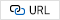Sungho Kim received a M.S. degree in electronics engineering from Inha University in 2020. He is currently working at Alphasolutions in Sungnam, Kyunggido, Korea. His research interests include high-speed interface circuit design for HDMI and MIPI.

Taek-Joon An received a B.S. and M.S. degree in electronics engi-neering from Inha University. He is currently pursuing an Ph.D. degree in Electrical and Computer Engineering. His research interests include CMOS high-speed interface circuit and clock and data recovery circuit design.

Yongwoo Kim received the B.S. and M.S. degrees from Inha University, Incheon, South Korea, in 2007 and 2009, respectively, and the Ph.D. degree in electrical engineering from the Korea Advanced Institute of Science and Technology, Daejeon, South Korea, in 2019., From 2009 to 2017, he was a Senior Engineer with LX Semicon Company Ltd., Daejeon. From 2019 to 2020, he was a Senior Researcher with the Artificial Intelligence Research Division, Korea Aerospace Research Institute, Daejeon. Since 2020, he has been with Sangmyung University, Cheonan, South Korea, where he is currently an Assistant Professor with the Department of System Semiconductor Engineering. His current research interests include image/video processing algorithms and deep learning hardware architecture for vision processing.

Jin-Ku Kang received the Ph.D. degree in electrical and computer engineering from North Carolina State University, Raleigh, NC, USA. From 1983 to 1988, he was with Samsung Electronics, Inc., South Korea. In 1988, he was with Texas Instruments, South Korea. From 1996 to 1997, he was with Intel Corp., Portland, OR, USA, as a senior design engineer, where he was involved in high-speed I/O and timing circuits for microprocessors. Since 1997, he has been with Inha University, Incheon, South Korea, where he is currently a professor and leads the System IC Design Laboratory in the Department of Electronics Engineering. His research interests include high-speed/low-power mixed-mode circuit design for high-speed serial interfaces.